<< Back to Off-topic Forum .GrayedOutTextBox { color: Gray; }

 Posts 1 - 16 of 16
Algebraic Rating of Warlight: 5/14/2017 11:57:18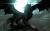Ashmage
Level 48
Report
Please represent your rating of Warlight in an algebraic expression in 1 to 10000. 10000 is the best.
Algebraic Rating of Warlight: 5/14/2017 12:18:32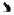Japanball
Level 56
Report
6921
Algebraic Rating of Warlight: 5/14/2017 14:04:16DanWL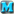Level 62
Report
9x - 15y = 1050
2x + 2y = 500

z = xy

Work out z (in number form).

Edited 5/14/2017 14:05:23
Algebraic Rating of Warlight: 5/14/2017 14:20:27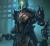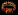OnlyThePie
Level 54
Report
z = 10000
Algebraic Rating of Warlight: 5/14/2017 16:24:34Japanball
Level 56
Report
9x-15y=1050
2x+2y=500

18x-30y=2100
18x+2y=4500

a+(b+32)=c+2400
a-b=c
2b+32=2400
2(30y)+32=2400
60y+32=2400
60y=2368
I went wrong somewhere
Algebraic Rating of Warlight: 5/14/2017 18:07:31OnlyThePie
Level 54
Report
2x=500-2y
x=250-y
9(250-y)-15y=1050
2250-9y-15y=1050
-24y=-1200
y=50
2x=2(50)=500
2x=400
x=200

z=(200)(50)
z=10000
Algebraic Rating of Warlight: 5/14/2017 19:03:51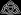apollong3
Level 53
Report
18x - 30y = 2100 (1)
-18x - 18y = -4500 (2)

(1)+(2) => -48y = -2400 => y= 50

(2) => 2x + 100 = 500 => 2x= 400 => x=200

z = 200*50 => z= 10000

When you multiply (2x + 2y = 500) with 9, remember to multiply everything with 9
Algebraic Rating of Warlight: 5/14/2017 19:32:04apollong3
Level 53
Report
If:

lnx -ln3.5 -ln2 +log(10^1/128) = 1/2^x (x > 0)

y=1000x

find y to reveal my rating

(note that e.g. x^? means "x" to the power of "?")

Edited 5/14/2017 19:32:37
Algebraic Rating of Warlight: 5/14/2017 23:10:44DanWLLevel 62
Report
a = (b+c)/c - (b+c) - 3c
b = 2c
(c/5) * √25 = 0.75 + 1/4
d = ((2b+c) - a) * 10^3
e = d/(4c) + d

Edit: typo - before e = d/(2c) + d

Edited 5/15/2017 09:47:55
Algebraic Rating of Warlight: 5/15/2017 02:06:53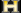Benjamin628Level 59
Report
x * [e^(pi*i)] = -10000

Edited 5/15/2017 02:07:21
Algebraic Rating of Warlight: 5/15/2017 06:16:47apollong3
Level 53
Report
so:
(1)=> lnx - (ln3.5 +ln2) -1/2^x = -log(10^1/128)

however:
ln3.5 +ln2 = ln(3.5*2) = ln7

and:
log(10^1/128) = 1/128 = (128 = 2^7) = 1/2^7

so:

lnx -1/2^x = ln7 -1/2^7 (3)

I now define a function f(x) = lnx -1/2^x

I will skip the proof, but just take it that f(x) is strictly increasing(that means that for every x,y that belong to (0, +infinity), with x < y , f(x) < f(y). This also means that: x = y <=> f(x) = f(y) )

(3) => f(x) = f(7) => x = 7

that means y = 7*1000 => y = 7000

EDIT:
http://prnt.sc/f7xz7t

eh, that's pretty much accurate

Edited 5/15/2017 06:47:31
Algebraic Rating of Warlight: 5/15/2017 07:18:08apollong3
Level 53
Report
That is indeed what I meant.
Algebraic Rating of Warlight: 5/15/2017 09:48:50DanWLLevel 62
Report
c = 1
b = 2
a = -3
d = 8000
e = 10000

Let me show how:
(c/5) * √25 = 0.75 + 1/4
(c/5) * 5 = 1
the divide and multiply cancel out...
c = 1

b = 2c
b = 2*1 = 2

a = (b+c)/c - (b+c) - 3c
a = (2+1)/1 - (2+1) - 3*1
a = 3/1 - 3 - 3
a = 3 - 3 - 3
a = 0 - 3
a = -3

d = ((2b+c) - a) * 10^3
d = ((2*2+1) - -3) * 1000
two negatives make a positive, so - -3 = +3
d = ((4+1) + 3) * 1000
d = (5+3) * 1000
d = 8 * 1000
d = 8000

e = d/(4c) + d
e = 8000/(4*1) + 8000
e = 8000/4 + 8000
e = 2000 + 8000
e = 10000
Algebraic Rating of Warlight: 5/15/2017 10:04:36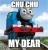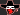KrzysztofLevel 66
Report
z = DanWL,
knowing that D = 44, anWL is equal to fat black man eating pizza with onion, strawberry and pudding, John F. Kennedy was assassinated by mad cow and Vladimir Putin is twin sister of Julius Ceasar - solve X
Algebraic Rating of Warlight: 5/15/2017 11:15:36DanWLLevel 62
Report
^
X >= 1 and X <= 10000
because this thread says that the number can only be between 1 and 10000, x is somewhere in between.
That's all you wanted me to solve

Solve (Krzysztof)/180540 to zero decimal places. The answer should be between or equal to 9000 and 10000.

K = f/25
r = tK (to two decimal places)
z = 4K ± 10
y = √42 (to two decimal places)
s = zy (to two decimal places)
t = oy - (z/2) (to two decimal places)
o = 2y (to two decimal places) ± 3
f = 5*-1

Edited 5/15/2017 13:32:33
Algebraic Rating of Warlight: 5/15/2017 12:02:20Ashmage
Level 48
Report
DanWL that's clever.
 Posts 1 - 16 of 16